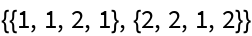#Function Repository Resource:

# ValidGraphColoring

Check if the given graph coloring is valid

Contributed by: Jongwan Kim
 ResourceFunction["ValidGraphColoring"][graph,coloring] gives True if no two adjacent vertices in graph have the same color as specified by coloring.

## Details and Options

In ResourceFunction["ValidGraphColoring"][graph,coloring], coloring can be a list of actual colors or numbers indicating distinct colors.
The value for graph must be an instance of a graph, not an adjacency matrix or list.

## Examples

### Basic Examples

Determine if the given coloring of a complete graph with two vertices is valid:

 In:=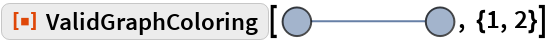Out=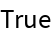### Applications

Find the valid colorings of a graph:

 In:=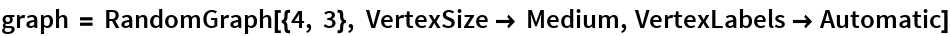Out=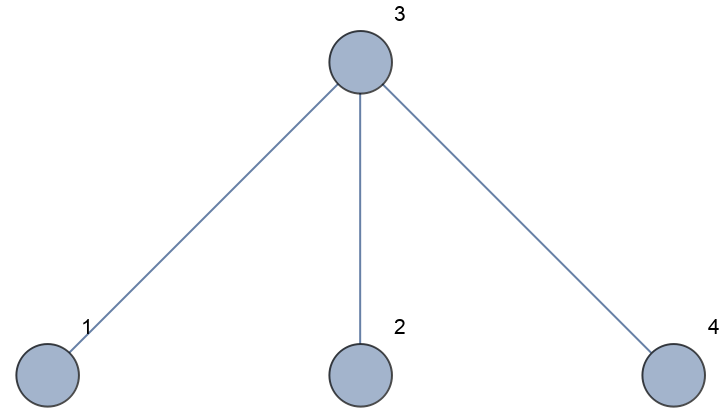Determine the minimum number of colors to color the graph:

 In:=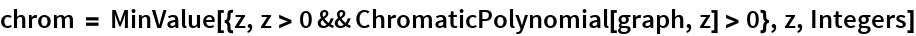Out=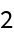In:=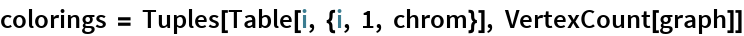Out=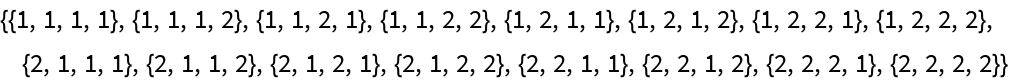In:=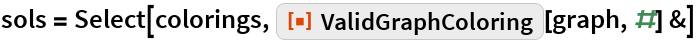Out=